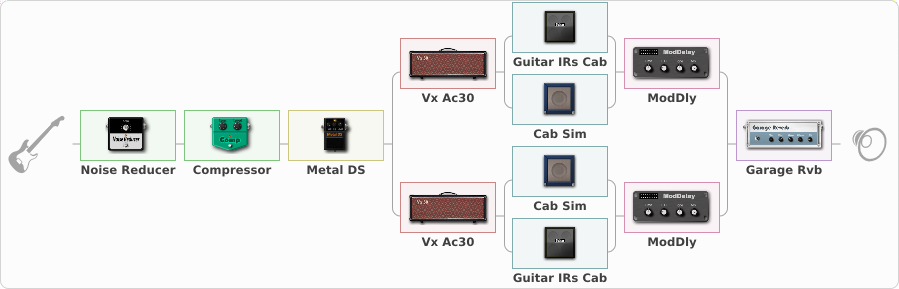# cricri83

Discussion in 'ToneLib-GFX presets' started by cricri83, Nov 6, 2021.

1. cricri83

Preset name: Style : Brian May (One Vision)

Effects chain:Effect: "Noise Reducer" (Dynamique / Filtre), active - "yes"
{
"Sens" = 80
"Mode" = Hard
}

Effect: "Compressor" (Dynamique / Filtre), active - "yes"
{
"Sense" = 39
"Level" = 80
}

Effect: "Metal DS" (Overdrive / Distortion), active - "yes"
{
"Dist" = 35
"Bass" = 40
"Middle" = 70
"Treble" = 80
"Level" = 60
}

Effect: "Splitter" (Dynamique / Filtre)
{
"A-Bypass" = Off
"A-Pan" = -100
"A-Level" = 65
"B-Bypass" = Off
"B-Pan" = 100
"B-Level" = 64

'A' branch:
{

Effect: "Vx Ac30" (Simulateurs d'amplis), active - "yes"
{
"Gain" = 50
"Bass" = 50
"Middle" = 80
"Treble" = 90
"Presence" = 25
"Master" = 80
"Level (dB)" = 2
}

Effect: "Splitter" (Dynamique / Filtre)
{
"A-Bypass" = Off
"A-Pan" = -98
"A-Level" = 55
"B-Bypass" = Off
"B-Pan" = 92
"B-Level" = 55

'A' branch:
{

Effect: "Guitar IRs Cab" (Cabinets), active - "yes"
{
"Model" = Vox AC30 (2x12")
"Mic Position" = Center
"Mic Distance" = Middle
"Low Cut (Hz)" = 60
"Hi Cut (kHz)" = 20.0
"Mix" = 80
"Level (dB)" = 0
}
}
'B' branch:
{

Effect: "Cab Sim" (Cabinets), active - "yes"
{
"Model" = 2x12" UK Blackback
"Level (dB)" = 2
}
}
}

Effect: "ModDly" (Retard), active - "yes"
{
"Time" = 280
"Feedback" = 25
"Tone" = 50
"Speed" = 1.6
"Mix" = 60
}
}
'B' branch:
{

Effect: "Vx Ac30" (Simulateurs d'amplis), active - "yes"
{
"Gain" = 50
"Bass" = 50
"Middle" = 80
"Treble" = 90
"Presence" = 25
"Master" = 80
"Level (dB)" = 2
}

Effect: "Splitter" (Dynamique / Filtre)
{
"A-Bypass" = Off
"A-Pan" = 100
"A-Level" = 55
"B-Bypass" = Off
"B-Pan" = -100
"B-Level" = 55

'A' branch:
{

Effect: "Cab Sim" (Cabinets), active - "yes"
{
"Model" = 2x12" UK Blackback
"Level (dB)" = 2
}
}
'B' branch:
{

Effect: "Guitar IRs Cab" (Cabinets), active - "yes"
{
"Model" = Vox AC30 (2x12")
"Mic Position" = Center
"Mic Distance" = Middle
"Low Cut (Hz)" = 60
"Hi Cut (kHz)" = 20.0
"Mix" = 80
"Level (dB)" = 0
}
}
}

Effect: "ModDly" (Retard), active - "yes"
{
"Time" = 280
"Feedback" = 25
"Tone" = 50
"Speed" = 1.6
"Mix" = 60
}
}
}

Effect: "Garage Rvb" (Réverbération), active - "yes"
{
"Time" = 5.2
"PreDelay" = 40
"LoDamp" = 10
"HiDamp" = 30
"Mix" = 50
}

Note: You will need to download and install the ToneLib-GFX software to use the preset.

File size:
1.4 KB
Views:
2,057